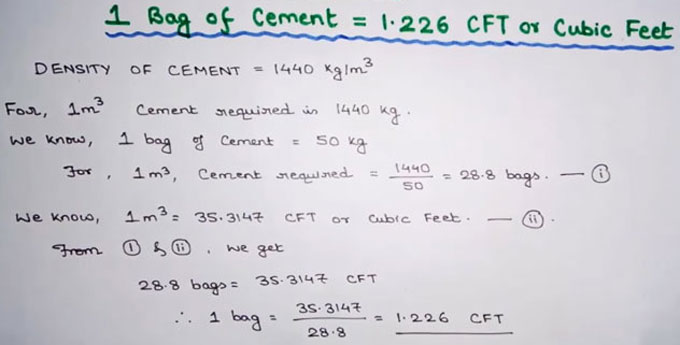# How to estimate cement bags in 1 cubic meterSuppose the proportion of nominal mix is 1:2:4 (one part cement, 2 part sand and 4 part aggregate)

Wastage of cement is taken as 2%
Output of mix is provided as 67%.

For 1 cum output, the requirement of dry mix is 1/0.67 = 1.49 say 1.50 cum.
After including the wastage (2%), the output will be (1.50 + 0.02) = 1.52 cum.
Volume of cement = (cement/cement+sand+aggregate) ? Total material

= (1/1+2+4) ? 1.52
=0.2171 cum
The density of cement is 1440 kg/cum and
Weight of 1 bag cement = 50 kg.

So, volume of 1 bag cement = 50/1440
=0.0347 cum.
No. of cement bags essential in 1 cubic meter = 0.2171/0.0347
= 6.25 bags.
The above formula can be utilized for measuring cement for other nominal mixes.

To get more details, watch the following video tutorial.

Video Source: Raj Kumar